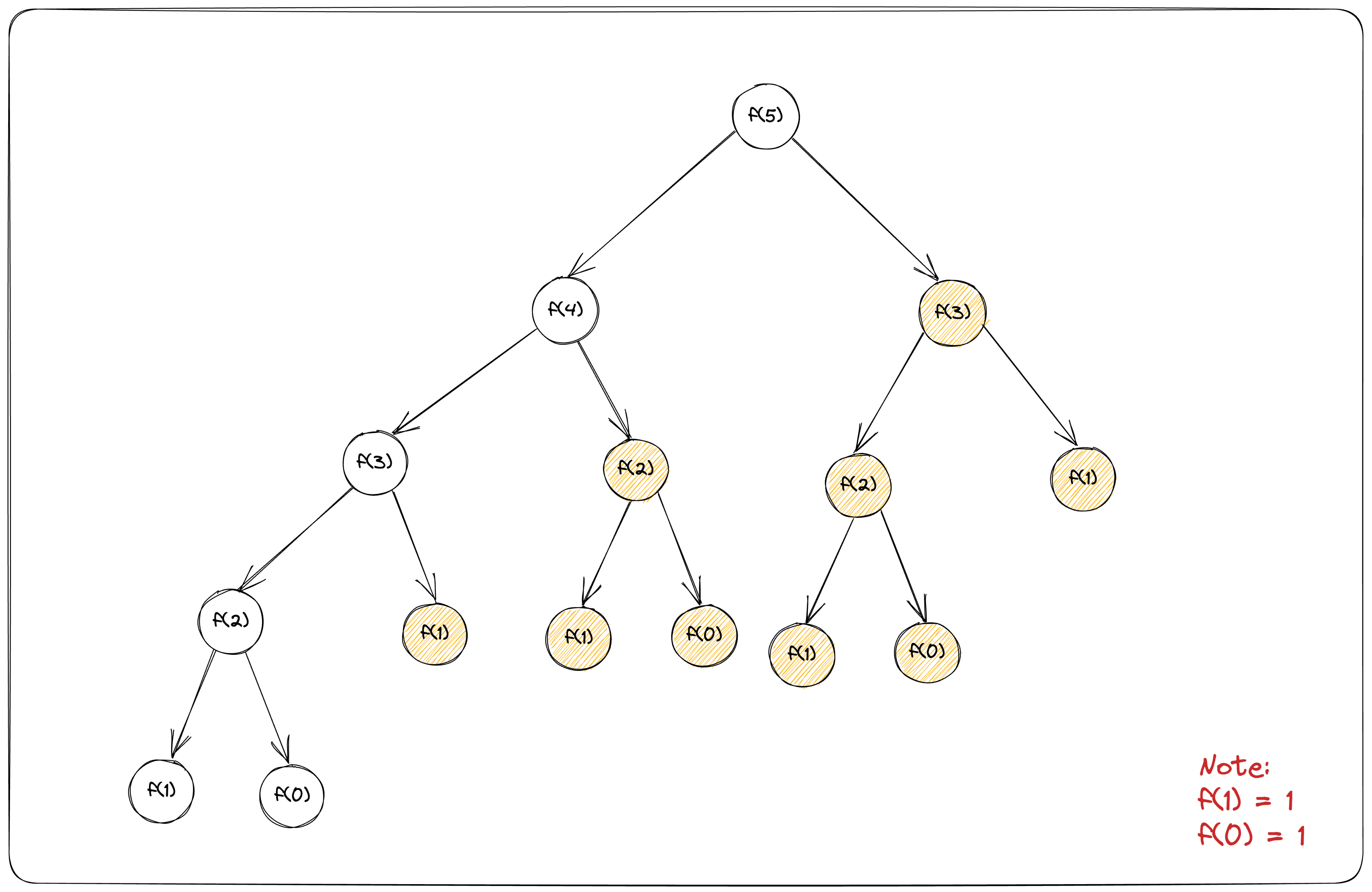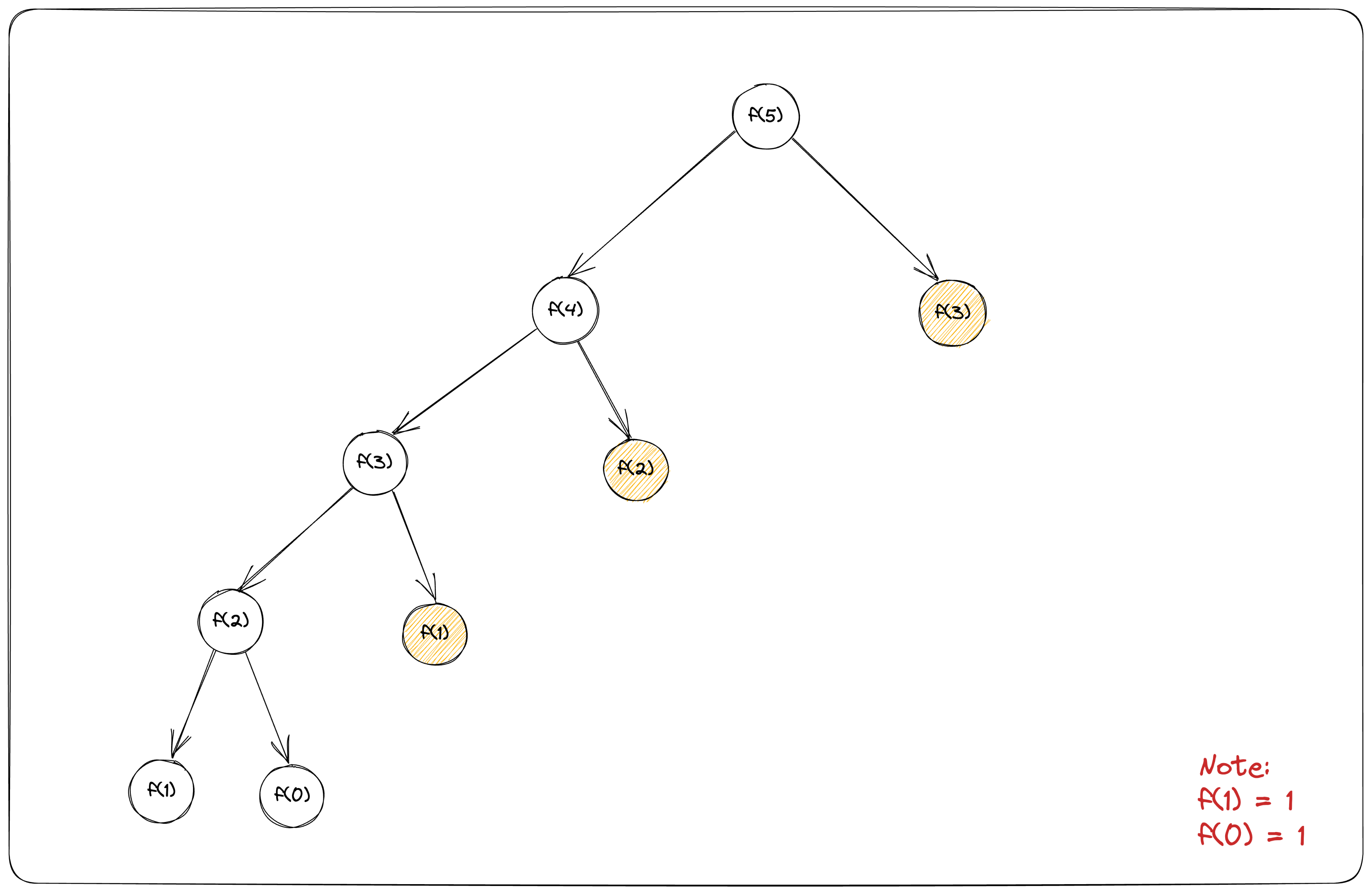# Understanding Dynamic Programming With JavaScriptHave you ever heard the phrase "work smarter, not harder"? Well, that's exactly what dynamic programming is all about!

Sometimes when you have a big problem to solve, you can make it smaller by breaking it down into pieces, then solve each piece one by one and finally put these solutions together. This is called the “divide and conquer" approach.

In this article, you'll learn what dynamic programming is, how it works, and how to use it to solve problems with JavaScript.

## What is Dynamic Programming?

Dynamic programming is a problem-solving technique that breaks down a complex problem into smaller, more manageable subproblems and then solves each subproblem only once and stores its solution. This technique is used in many fields, such as mathematics, economics, and computer science.

Imagine that you have a big puzzle to solve. Instead of trying to solve the whole puzzle at once, you could break it down into smaller, more manageable pieces and solve each one individually. This is exactly what dynamic programming does.

One way to understand dynamic programming is by looking at the Fibonacci sequence. The Fibonacci sequence is a series of numbers where each number is the sum of the two preceding numbers. For example, the sequence goes like this: 0, 1, 1, 2, 3, 5, 8, 13, 21, 34, and so on.

Let's say you want to find the 5th number in the Fibonacci sequence. Then, you need to calculate the 4th and 3rd numbers in the sequence. To calculate the 4th number, you need to add the 2nd and 3rd numbers together. And it continues that way.But you’ll notice there is a lot of repetition. f(3), which stands for fibonacci(2) or 2nd fibonacci will be calculated thrice — that’s a lot of calculations for one problem! What if you need to find the 100th number in the sequence? This is where dynamic programming comes in.

Instead of re-calculating the same numbers repeatedly, you can use memoization to store the results of previous calculations in a special memory. So, when you need to find a number in the sequence, you can check if you've already calculated it before and just use the result stored in memory. This makes your calculations much faster and saves a lot of time!In the illustration above, the shaded nodes use memoized values instead of having to recalculate them.

This is just a simple example, but dynamic programming can be used to solve much more complex problems in a similar way by breaking them down into smaller, simpler problems and using memoization to store the results.

## Steps to Solve Dynamic Programming Problems

Here are the general steps to follow when solving Dynamic Programming problems:

• Define the problem in terms of subproblems: The first step is to identify the problem and determine if it can be broken down into smaller subproblems. Once you have identified the subproblems, you need to determine how to combine the solutions to these subproblems to obtain the solution to the original problem.

• Determine the recurrence relation: The next step is to determine the recurrence relation, which is a mathematical equation that relates the solution to a given subproblem to the solutions of smaller subproblems. This equation should express the solution to the problem in terms of solutions to smaller subproblems.

• Implement the solution using memoization: After determining the recurrence relation, you can implement the solution using memoization.

## How to Use Dynamic Programming with JavaScript

JavaScript is a programming language that is widely used for web development. One of the advantages of JavaScript is its flexibility, which makes it an excellent choice for implementing dynamic programming.

Let's see how you can implement dynamic programming in JavaScript using memoization. Memoization is a way of caching function results so that you can reuse them later without recalculating them.

For example, let's say you have a function to calculate the Fibonacci sequence for a given number:

``````function fibonacci(n) {
if (n <= 1) return n;
return fibonacci(n - 1) + fibonacci(n - 2);
}
``````

This function uses recursion to calculate the Fibonacci sequence. However, it calculates the same Fibonacci numbers multiple times, which can be inefficient for large values of `n`. For example, to calculate `fibonacci(5)`, it first calculates `fibonacci(4)` and `fibonacci(3)`, and to calculate `fibonacci(4)`, it calculates `fibonacci(3)` again. This is redundant.

To avoid this redundancy, you can use memoization. You can store the result of each Fibonacci calculation in an object and reuse it later if you need to calculate the same Fibonacci again.

Here's how you can implement memoization for the Fibonacci function:

``````function fibonacci(n, memo = {}) {
if (n in memo) return memo[n];
if (n <= 1) return n;
memo[n] = fibonacci(n - 1, memo) + fibonacci(n - 2, memo);
return memo[n];
}
``````

The function takes in a parameter `n` which specifies the index of the Fibonacci number to be computed. The second parameter `memo` is an object that stores the results of previously computed Fibonacci numbers so that they can be reused in later calculations.

The function first checks if the value of `n` already exists in the `memo` object. If it does, the function returns that value instead of recomputing it. This is the memoization technique. Next, the function checks if `n` is less than or equal to 1. If it is, it returns the value of `n`. This is because the first two numbers in the Fibonacci sequence are 0 and 1.

Finally, if the value of `n` is not in the `memo` object and it is greater than 1, the function recursively calls itself twice, passing in `n - 1` and `n - 2` as parameters respectively. The results of these two recursive calls are added together and stored in the `memo` object. The result is then returned.

## Conclusion

In this article, you have learned what dynamic programming is all about, where it’s useful and the various steps to implementing dynamic programming in your program.

Have fun coding!### Written by Joel Olawanle

Joel is a software engineer and teacher. He write's about software development, productivity, and life. He has written over 150 technical content that has helped millions of people all over the world.